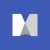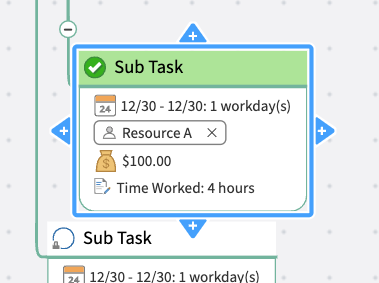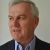# Time tracking on certain tasks

Nylah . shared this question ago

Hello,

Is it possible to track the amount of hours you worked on a particular task or sub/topic?Hi Nylah,

We don't have a specific feature to track hours, but you could add a note to record it. Here's an example:## Replies (2)1

Hi Nylah,

We don't have a specific feature to track hours, but you could add a note to record it. Here's an example:1

A topic property rather than a note might be a better option to record the number of hours worked, especially if you want to total the number of hours across tasks or perform other calculations.

Another option would be to use a formula to calculate the number of hours worked as a proportion of the total number of days allocated to the task based on progress. Of course this approach works only if the level of progress matches the hours actually worked to date.1

To clarify the second option I proposed, the formula based on progress could be used with either duration or effort.

A third option is use the Task Days Elapsed feature in the MindManager MAP add-in. This creates a topic property which shows the number of days elapsed since the task began. If the duration and effort are equal you only need to create a simple formula to multiply Task Days Elapsed by the number of hours worked per day.

If however they are different and you want to calculate the number of hours worked as pro rata to the effort expended in the time elapsed you would need to divide the Task Days Elapsed by the Duration in days, then multiply the result by the number of effort days and then by the number of hours worked per day. Again, this assumes that an even amount of effort is expended each day.

---